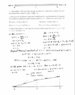# Heat Equation Problem

• I
FAS1998
I’ve attached an image of a solved problem related to the heat equation.

Can somebody explain the -c + 3d = 0 comes from? I’m having trouble following the work shown.

#### Attachments

•660AF30D-BD5E-4AC0-ABD5-D3DB8811F936.jpeg
43.9 KB · Views: 315

Perfect thermal contact occurs at x=1, so the flow coming from the right is equal to the flow coming from the left. Now recall that the flow is given by ##\phi=-K_0\frac{\partial u}{\partial x}##, that is Fourier's law. The derivatives on both sides are constants as it's shown on your work so we're just left with: $$K_{1}\dfrac{\partial u_1}{\partial x} = K_{2}\dfrac{\partial u_2}{\partial x} \\ -c = -3d \\ -c+3d=0$$ I took the liberty to introduce some notation since it's a little confusing in your solution, but essentially all you have to do is substitute the conductivities given in the exercise and the solutions you found for u.
•